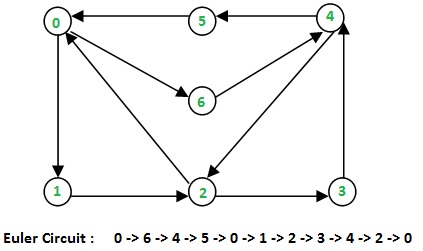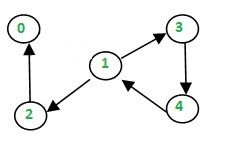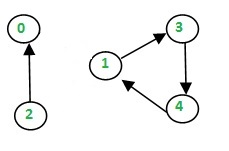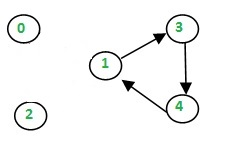# Hierholzer’s Algorithm for directed graph

• Difficulty Level : Medium
• Last Updated : 20 Apr, 2022

Given a directed Eulerian graph, print an Euler circuit. Euler circuit is a path that traverses every edge of a graph, and the path ends on the starting vertex.

Examples:

```Input : Adjacency list for the below graphOutput : 0 -> 1 -> 2 -> 0

Input : Adjacency list for the below graphOutput : 0 -> 6 -> 4 -> 5 -> 0 -> 1
-> 2 -> 3 -> 4 -> 2 -> 0
Explanation:
In both the cases, we can trace the Euler circuit
by following the edges as indicated in the output.
```

## Recommended: Please try your approach on {IDE} first, before moving on to the solution.

We have discussed the problem of finding out whether a given graph is Eulerian or not. In this post, an algorithm to print the Eulerian trail or circuit is discussed. The same problem can be solved using Fleury’s Algorithm, however, its complexity is O(E*E). Using Hierholzer’s Algorithm, we can find the circuit/path in O(E), i.e., linear time.

Below is the Algorithm: ref ( wiki ). Remember that a directed graph has a Eulerian cycle if the following conditions are true (1) All vertices with nonzero degrees belong to a single strongly connected component. (2) In degree and out-degree of every vertex is the same. The algorithm assumes that the given graph has a Eulerian Circuit.

• Choose any starting vertex v, and follow a trail of edges from that vertex until returning to v. It is not possible to get stuck at any vertex other than v, because indegree and outdegree of every vertex must be same, when the trail enters another vertex w there must be an unused edge leaving w.
The tour formed in this way is a closed tour, but may not cover all the vertices and edges of the initial graph.
• As long as there exists a vertex u that belongs to the current tour, but that has adjacent edges not part of the tour, start another trail from u, following unused edges until returning to u, and join the tour formed in this way to the previous tour.

Thus the idea is to keep following unused edges and removing them until we get stuck. Once we get stuck, we backtrack to the nearest vertex in our current path that has unused edges, and we repeat the process until all the edges have been used. We can use another container to maintain the final path.

Let’s take an example:

```Let the initial directed graph be as belowLet's start our path from 0.
Thus, curr_path = {0} and circuit = {}
Now let's use the edge 0->1Now, curr_path = {0,1} and circuit = {}
similarly we reach up to 2 and then to 0 again asNow, curr_path = {0,1,2} and circuit = {}
Then we go to 0, now since 0 haven't got any unused
edge we put 0 in circuit and back track till we find
an edgeWe then have curr_path = {0,1,2} and circuit = {0}
Similarly, when we backtrack to 2, we don't find any
unused edge. Hence put 2 in the circuit and backtrack
again.

curr_path = {0,1} and circuit = {0,2}

After reaching 1 we go to through unused edge 1->3 and
then 3->4, 4->1 until all edges have been traversed.

The contents of the two containers look as:
curr_path = {0,1,3,4,1} and circuit = {0,2}

now as all edges have been used, the curr_path is
popped one by one into the circuit.
Finally, we've circuit = {0,2,1,4,3,1,0}

We print the circuit in reverse to obtain the path followed.
i.e., 0->1->3->4->1->1->2->0
```

Below is the implementation for the above approach:

## C++

 `// A C++ program to print Eulerian circuit in given``// directed graph using Hierholzer algorithm``#include ``using` `namespace` `std;`` ` `void` `printCircuit(vector< vector<``int``> > adj)``{``    ``// adj represents the adjacency list of``    ``// the directed graph``    ``// edge_count represents the number of edges``    ``// emerging from a vertex``    ``unordered_map<``int``,``int``> edge_count;`` ` `    ``for` `(``int` `i=0; i curr_path;`` ` `    ``// vector to store final circuit``    ``vector<``int``> circuit;`` ` `    ``// start from any vertex``    ``curr_path.push(0);``    ``int` `curr_v = 0; ``// Current vertex`` ` `    ``while` `(!curr_path.empty())``    ``{``        ``// If there's remaining edge``        ``if` `(edge_count[curr_v])``        ``{``            ``// Push the vertex``            ``curr_path.push(curr_v);`` ` `            ``// Find the next vertex using an edge``            ``int` `next_v = adj[curr_v].back();`` ` `            ``// and remove that edge``            ``edge_count[curr_v]--;``            ``adj[curr_v].pop_back();`` ` `            ``// Move to next vertex``            ``curr_v = next_v;``        ``}`` ` `        ``// back-track to find remaining circuit``        ``else``        ``{``            ``circuit.push_back(curr_v);`` ` `            ``// Back-tracking``            ``curr_v = curr_path.top();``            ``curr_path.pop();``        ``}``    ``}`` ` `    ``// we've got the circuit, now print it in reverse``    ``for` `(``int` `i=circuit.size()-1; i>=0; i--)``    ``{``        ``cout << circuit[i];``        ``if` `(i)``           ``cout<<``" -> "``;``    ``}``}`` ` `// Driver program to check the above function``int` `main()``{``    ``vector< vector<``int``> > adj1, adj2;`` ` `    ``// Input Graph 1``    ``adj1.resize(3);`` ` `    ``// Build the edges``    ``adj1.push_back(1);``    ``adj1.push_back(2);``    ``adj1.push_back(0);``    ``printCircuit(adj1);``    ``cout << endl;`` ` `    ``// Input Graph 2``    ``adj2.resize(7);``    ``adj2.push_back(1);``    ``adj2.push_back(6);``    ``adj2.push_back(2);``    ``adj2.push_back(0);``    ``adj2.push_back(3);``    ``adj2.push_back(4);``    ``adj2.push_back(2);``    ``adj2.push_back(5);``    ``adj2.push_back(0);``    ``adj2.push_back(4);``    ``printCircuit(adj2);`` ` `    ``return` `0;``}`

## Python3

 `# Python3 program to print Eulerian circuit in given``# directed graph using Hierholzer algorithm``def` `printCircuit(adj):`` ` `    ``# adj represents the adjacency list of``    ``# the directed graph``    ``# edge_count represents the number of edges``    ``# emerging from a vertex``    ``edge_count ``=` `dict``()`` ` `    ``for` `i ``in` `range``(``len``(adj)):`` ` `        ``# find the count of edges to keep track``        ``# of unused edges``        ``edge_count[i] ``=` `len``(adj[i])`` ` `    ``if` `len``(adj) ``=``=` `0``:``        ``return` `# empty graph`` ` `    ``# Maintain a stack to keep vertices``    ``curr_path ``=` `[]`` ` `    ``# vector to store final circuit``    ``circuit ``=` `[]`` ` `    ``# start from any vertex``    ``curr_path.append(``0``)``    ``curr_v ``=` `0` `# Current vertex`` ` `    ``while` `len``(curr_path):`` ` `        ``# If there's remaining edge``        ``if` `edge_count[curr_v]:`` ` `            ``# Push the vertex``            ``curr_path.append(curr_v)`` ` `            ``# Find the next vertex using an edge``            ``next_v ``=` `adj[curr_v][``-``1``]`` ` `            ``# and remove that edge``            ``edge_count[curr_v] ``-``=` `1``            ``adj[curr_v].pop()`` ` `            ``# Move to next vertex``            ``curr_v ``=` `next_v`` ` `        ``# back-track to find remaining circuit``        ``else``:``            ``circuit.append(curr_v)`` ` `            ``# Back-tracking``            ``curr_v ``=` `curr_path[``-``1``]``            ``curr_path.pop()`` ` `    ``# we've got the circuit, now print it in reverse``    ``for` `i ``in` `range``(``len``(circuit) ``-` `1``, ``-``1``, ``-``1``):``        ``print``(circuit[i], end ``=` `"")``        ``if` `i:``            ``print``(``" -> "``, end ``=` `"")`` ` `# Driver Code``if` `__name__ ``=``=` `"__main__"``:`` ` `    ``# Input Graph 1``    ``adj1 ``=` `[``0``] ``*` `3``    ``for` `i ``in` `range``(``3``):``        ``adj1[i] ``=` `[]`` ` `    ``# Build the edges``    ``adj1[``0``].append(``1``)``    ``adj1[``1``].append(``2``)``    ``adj1[``2``].append(``0``)``    ``printCircuit(adj1)``    ``print``()`` ` `    ``# Input Graph 2``    ``adj2 ``=` `[``0``] ``*` `7``    ``for` `i ``in` `range``(``7``):``        ``adj2[i] ``=` `[]`` ` `    ``adj2[``0``].append(``1``)``    ``adj2[``0``].append(``6``)``    ``adj2[``1``].append(``2``)``    ``adj2[``2``].append(``0``)``    ``adj2[``2``].append(``3``)``    ``adj2[``3``].append(``4``)``    ``adj2[``4``].append(``2``)``    ``adj2[``4``].append(``5``)``    ``adj2[``5``].append(``0``)``    ``adj2[``6``].append(``4``)``    ``printCircuit(adj2)``    ``print``()`` ` `# This code is contributed by``# sanjeev2552`

Output:

```0 -> 1 -> 2 -> 0
0 -> 6 -> 4 -> 5 -> 0 -> 1 -> 2 -> 3 -> 4 -> 2 -> 0
```

Alternate Implementation :
Below are the improvements made from the above code
* The above code kept a count of the number of edges for every vertex. This is unnecessary since we are already maintaining the adjacency list. We simply deleted the creation of edge_count array. In the algorithm we replaced `if edge_count[current_v]` with `if adj[current_v]`
* The above code pushes the initial node twice to the stack. Though the way he coded the result is correct, this approach is confusing and inefficient. We eliminated this by appending the next vertex to the stack, instead of the current one.
* In the main part, where the author tests the algorithm, the initialization of the adjacency lists `adj1` and `adj2`were a little weird. That potion is also improved.

## Python3

 `# Python3 program to print Eulerian circuit in given``# directed graph using Hierholzer algorithm``def` `printCircuit(adj):``  ` `    ``# adj represents the adjacency list of``    ``# the directed graph``      ` `    ``if` `len``(adj) ``=``=` `0``:``        ``return` `# empty graph``  ` `    ``# Maintain a stack to keep vertices``    ``# We can start from any vertex, here we start with 0``    ``curr_path ``=` `[``0``]``  ` `    ``# list to store final circuit``    ``circuit ``=` `[]``  ` `    ``while` `curr_path:``  ` `        ``curr_v ``=` `curr_path[``-``1``]``          ` `        ``# If there's remaining edge in adjacency list  ``        ``# of the current vertex ``        ``if` `adj[curr_v]:`` ` `            ``# Find and remove the next vertex that is  ``            ``# adjacent to the current vertex``            ``next_v ``=` `adj[curr_v].pop()``  ` `            ``# Push the new vertex to the stack``            ``curr_path.append(next_v)``  ` `        ``# back-track to find remaining circuit``        ``else``:``            ``# Remove the current vertex and ``            ``# put it in the circuit``            ``circuit.append(curr_path.pop())``  ` `    ``# we've got the circuit, now print it in reverse``    ``for` `i ``in` `range``(``len``(circuit) ``-` `1``, ``-``1``, ``-``1``):``        ``print``(circuit[i], end ``=` `"")``        ``if` `i:``            ``print``(``" -> "``, end ``=` `"")``  ` `# Driver Code``if` `__name__ ``=``=` `"__main__"``:``  ` `    ``# Input Graph 1``    ``adj1 ``=` `[[] ``for` `_ ``in` `range``(``3``)]``  ` `    ``# Build the edges``    ``adj1[``0``].append(``1``)``    ``adj1[``1``].append(``2``)``    ``adj1[``2``].append(``0``)``    ``printCircuit(adj1)``    ``print``()``  ` `    ``# Input Graph 2``    ``adj2 ``=` `[[] ``for` `_ ``in` `range``(``7``)]``  ` `    ``adj2[``0``].append(``1``)``    ``adj2[``0``].append(``6``)``    ``adj2[``1``].append(``2``)``    ``adj2[``2``].append(``0``)``    ``adj2[``2``].append(``3``)``    ``adj2[``3``].append(``4``)``    ``adj2[``4``].append(``2``)``    ``adj2[``4``].append(``5``)``    ``adj2[``5``].append(``0``)``    ``adj2[``6``].append(``4``)``    ``printCircuit(adj2)``    ``print``()`

Output:

```0 -> 1 -> 2 -> 0
0 -> 6 -> 4 -> 5 -> 0 -> 1 -> 2 -> 3 -> 4 -> 2 -> 0
```

Time Complexity : O(V+E).

This article is contributed by Ashutosh Kumar. The article contains also inputs from Nitish Kumar. If you like GeeksforGeeks and would like to contribute, you can also write an article using write.geeksforgeeks.org or mail your article to review-team@geeksforgeeks.org. See your article appearing on the GeeksforGeeks main page and help other Geeks.